Module 9 Risk Management & Trading Psychology

Chapter 3

# Risk (Part 2) – Variance & Covariance

## 3.1 – VarianceIn the previous chapter, we touched upon the topic of expected return, continuing on it, we will understand the concept of ‘Portfolio variance’. Portfolio Variance helps us understand the risk at a portfolio level. I’m hoping you are familiar with ‘Standard Deviation’ as a measure of risk. We have discussed standard deviation multiple times in the previous modules (refer to Module 5, chapter 15 onwards). I’d suggest you get familiar with it if you are not already. While we can easily measure the risk of a single stock by calculating its standard deviation, calculating the risk of a portfolio is a whole different ball game. When you put a few individual stocks together and create a portfolio, it becomes a different animal altogether. The agenda for this chapter is to help you understand how to estimate risk at a portfolio level.

However, before we proceed, we need to understand the concept of Variance and Covariance. Both Variance and Covariance are statistical measures. Let’s deal with the Variance first.

The variance of stock returns is a measure of how much a stock’s return varies with respect to its average daily returns. The formula to calculate variance is quite straight forward –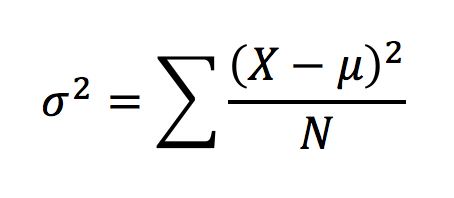Where,

σ2 = Variance

X = Daily return

µ = Average of daily return

N = Total number of observation

Note, the variance is measured as sigma squared; I will not get into the reasons for this as the explanation is quite complex and we could digress. For now, I’d request you to be aware of the fact that variance is sigma squared. Anyway, calculating variance is quite simple, I’ll take a simple example to help us understand this better.

Assume the daily return for a stock for 5 consecutive days is as below –

Day 1 – + 0.75%

Day 2 – + 1.25%

Day 3 – -0.55%

Day 4 – -0.75%

Day 5 – +0.8%.

In this case, the average return is +0.3%. We now need to calculate the dispersion of daily return over its average return, and also square the dispersion.

Daily Return Dispersion from average Dispersion squared
+ 0.75% 0.75% – 0.3% = + 0.45% 0.45%^2 = 0.002025%
+ 1.25% +1.25% – 0.3% = + 0.95% 0.95%^2 = 0.009025%
-0.55% -0.55% – 0.3% = -0.85% -0.85%^2 = 0.007225%
-0.75% -0.75% – 0.3% = -1.05% -1.05%^2 = 0.011025%
+0.80% +0.8% – 0.3% = +0.5% 0.50%^2 = 0.002500%

We now sum up the dispersion squared to get 0.0318000%. We divide this over 5 (N) to get the variance i.e

0.0318000% / 5

σ= 0.0063600%.

So what does this number tell us? It gives us a sense of how the daily returns are spread out from the average expected returns. So you as an investor should look into the variance to determine the riskiness of the investment. A large variance indicates that the stock could be quite risky while a small variance can indicate lesser risk. In the above example, I would consider the variance high, since we are looking at just 5 days worth of data.

Now, here is something you may be interested in knowing. Variance and standard deviation are related to each other by the following simple mathematical relationship –

Square Root of Variance = Standard Deviation

We can apply this to the example above and calculate the 5-day standard deviation of the stock,

%

~ 0.8%

which is the standard deviation a.k.a. the volatility of the stock (over the last 5 days). Anyway, at this point, I want you to be aware of Variance and what it really means. We will eventually plug variance along with covariance into the portfolio variance equation.

## 3.2 – Covariance

Covariance indicates how two (or more) variables move together. It tells us whether the two variables move together (in which case they share a positive covariance) or they move in the opposite direction (negatively covariance).  Covariance in the context of stock market measures how the stock prices of two stocks (or more) move together.  The two stocks prices are likely to move in the same direction if they have a positive covariance; likewise, a negative covariance indicates that they two stocks move in opposite direction.

I understand covariance may sound similar to ‘correlation’, however, the two are different. We will discuss more on this further in the chapter.

I guess calculating the covariance for two stocks will help us get a grip on understanding covariance better. The formula to calculate covariance of two stocks is as follows –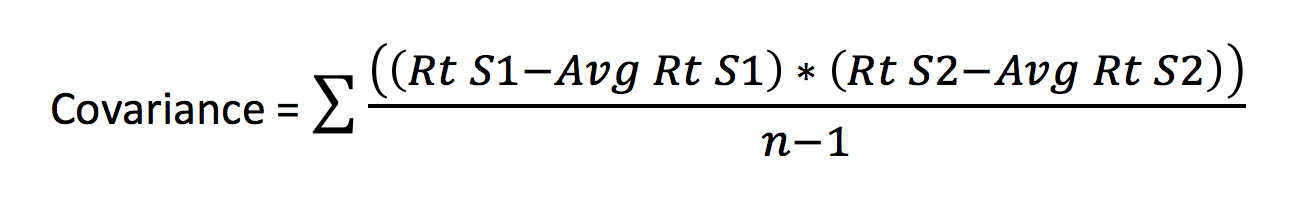Where,

Rt S1 = Daily stock return of stock 1

Avg Rt S1 = Average return of stock 1 over n period

Rt S2 = Daily stock return of stock 2

Avg Rt S2 = Average return of stock 2 over n period

n – The total number of days

In other words, you can calculate the covariance between two stocks by taking the sum product of the difference between the daily returns of the stock and its average return across both the stocks.

Sounds confusing? I guess so. ☺

Let us take up an example and see how we can calculate the covariance between two stocks.

For the sake of this illustration, I’ve selected two stocks – Cipla Limited and Idea Cellular Limited. To calculate the covariance between these two stocks, we need to work around with the above formula. We will resort to good old excel to help us implement the formula.

Before we proceed, if you were to guess the covariance between Cipla and Idea, what do you think it would be? Think about it – two large corporate, similar size, but in two completely unrelated sectors. What do you think would be the covariance? Give it a thought.

Anyway, here are the steps involved in calculating covariance in excel (note, although there is a direct function in excel to calculate covariance, I’ll take the slightly longer approach, just to ensure clarity) –

Step 1 – Download the daily stock prices. For the purpose of this illustration, I’ve downloaded 6 months data for both the stocks.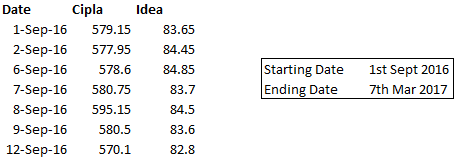Step 2 – Calculate the daily returns for both the stocks. Daily returns can be calculated by dividing today’s stock price over yesterday’s stock price and subtracting 1 from the result of this division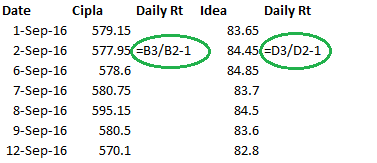Step 3 – Calculate the average of the daily returns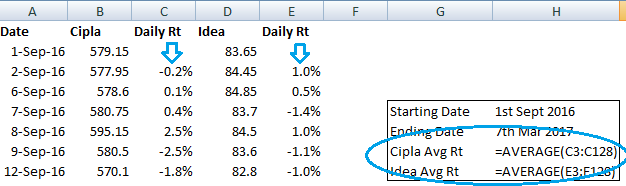Step 4 – Once the average is calculated, subtract the daily return by its average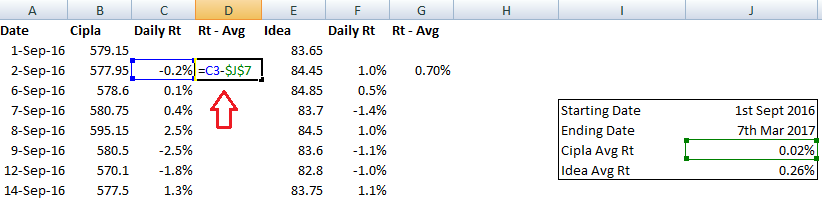Step 5 – Multiply the two series calculated in the previous step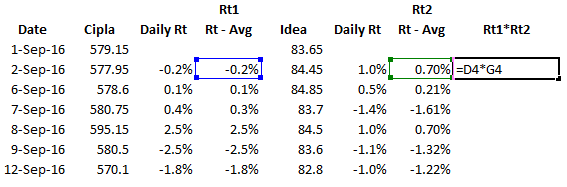Step 6 – Sum up the calculation made in the previous step. Take a count of the number of data points. You can do this by using the count function in excel and giving any of the fields as the input array. I’ve used the count on the dates here.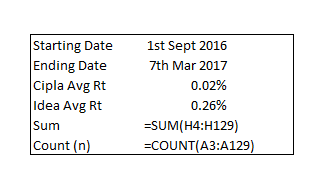Step 7 – This is the final step in calculating the covariance. To do so, one needs to divide the sum by count minus 1 i.e (n-1). The count, in this case, is 127, so count-1 would be 126. Sum calculated in the previous step was 0.006642. Hence, covariance would be

= 0.006642/126

= 0.00005230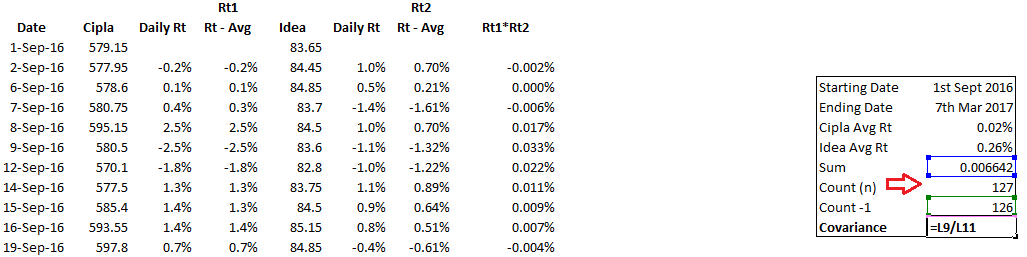As you can see, the covariance number is quite small. However, that’s not the point here. We only look at whether the two stocks share a positive or negative covariance. Clearly, since the two stocks share a positive covariance, it means that the returns of the two stocks move in similar directions. It means that for a given situation in the market, both the stocks are likely to move in the same direction. Note – covariance does not tell us the degree to which the two stocks move. The degree or magnitude is captured by correlation. The correlation between Idea and Cipla is 0.106, which indicates that the two stocks are not tightly correlated.

By the way, here is something very interesting fact. The mathematical equation for correlation between two stocks is as follows –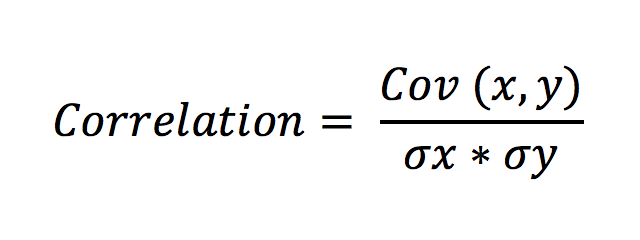Where,

Cov (x,y) is the covariance between the two stocks

σx = Standard deviation of stock x

σy = Standard deviation of stock y

Note, the standard deviation of a stock is simply the square root of the variance of the stock. Here is a task for you – we have calculated the correlation between Idea and Cipla using the direct excel function. Can you confirm the accuracy by implementing the formula?

Anyway, in the case of building a stock portfolio, do you think a positive covariance is good or bad? Or rather do portfolio managers desire stocks (in their portfolio) which share a positive covariance or they don’t? Well, portfolio managers strive to select stocks which share a negative covariance. The reason is quite simple – they want stocks in the portfolio which can hold up. Meaning if one stock goes down, they want, at least the other to hold up. This kind of counter balances the portfolio and reduces the overall risk.

Now, think about a regular portfolio – it will certainly contain more than 2 stocks. In fact, a good portfolio will contain at least 12-15 stocks. How would one measure covariance in this case? This is where things start getting complicated. One will have to measure covariance of each stock with all the other stocks in the portfolio. Let me illustrate this with a 4 stocks portfolio. Assume the portfolio is like this –

1. ABB
2. Cipla
3. Idea
4. Wipro

In this case, we need to calculate the covariance across –

1. ABB, Cipla
2. ABB, Idea
3. ABB, Wipro
4. Cipla, Idea
5. Cipla, Wipro
6. Idea, Wipro

Note, the covariance between stock 1 and stock 2 is the same as the covariance between stock 2 and stock 1. So as you can see, 4 stocks require us to compute 6 covariances. You can imagine the complexity when we have 15 or 20 stocks. In fact, when we have more than 2 stocks in the portfolio, the covariance between them is calculated and tabulated using a ‘Variance – Covariance Matrix’. I would love to talk about this now, but I guess, I’ll will keep it for the next chapter.

Stay tuned for more!

### Key takeaways from this chapter

1. Variance measures the dispersion of returns over the expected average returns
2. Higher variance indicates higher risk, lower variance indicates lower risk
3. Square root of variance is standard deviation
4. Covariance between the returns of two stock measures how the returns of the two stocks vary
5. A positive covariance indicates that the returns move positively and a negative covariance indicates that while one stock returns moves up, the other comes down
6. Correlation measures the strength of the movement
7. Covariance between two stocks divided over their individual standard deviations results in a correlation between two stocks.
8. When we have more than 2 stocks in a portfolio, we compute the variance-covariance using a matrix
Module 9

#### Chapters

1.Susim Samanta says:

I have calculated variance of stock using daily return of last 1000 days. Then I calculated Standard Deviation using square root of variance.Then I calculated Standard Deviation using standard deviation method. But values are not matching. Do you know why? e.g I took “NSE:ITC” everyday close value of past 1000 days. So from variance calculations I am getting “0.000487” and from Standard Deviation method I am getting “0.1788465”. Am I doing anything wrong calculations?

•Karthik Rangappa says:

Need to check the excel for this, ideally they should match.

•susim says:

•Karthik Rangappa says:

Will look into this shortly.

•susim says:

Thanks! Did you get a chance to look into this one? Thanks in advance.

•Karthik Rangappa says:

Not yet, Susim. I’ve been really tied up with few things.

•susim says:

no problem thanks!

2.Sai Sreedhar says:

Bravo!
Is it possible that covariance could change over a long period for any 2 (or more) stocks? Supposing it was positive when we buy them and it might becomes negative at some point of the holding period (or vice versa)

•Karthik Rangappa says:

Over a long time, yes, there is a chance for covariance to change.

3.kavya says:

Shouldn’t we consider the trading holidays for such calculations. Since stocks only moves on live trading session. So average daily returns will not be whole 365 days but actual trading days. Just a smart doubt.

•Karthik Rangappa says:

Yes, on trading holidays we wont have any data points anyway.

4.Yoganantham Gunasekaran says:

Hi karthik, Is there anyway to download last 10 year data of Nifty in a single excel file from NSE website. Right now I can able to download only one year data at a time.

•Karthik Rangappa says:

No, NSE used to allow that few years ago but have stopped doing so.

•YOGANANTHAM GUNASEKARAN says:

By the way thanks for this great initiative(varsity) by your team. Looking forward for Trading strategies and system

5.Ramaraj says:

Hello Varsity team, I have made complete Varsity documents as audio book ( Uploaded in You tube channel). Where i need your confirmation/approval to publish as education content.

•Karthik Rangappa says:

•Ramaraj says:

Thanks Karthik , I do share the link once all videos uploaded.

•Karthik Rangappa says:

Sure, thank you so much.

6.intelharsh says:

Hello Kartik,
Can you have pdf or ipub format for chapter no 8.? I want to download those entire chapters.

•Karthik Rangappa says:

We are working on it.

7.Ramaraj says:

Hello Karthik

Here is the play list where all video included

•Karthik Rangappa says:

Thanks Ramaraj. Good luck and all the very best.

•AD says:

Ramaraj,
Haven’t seen the videos yet but it is nice on your part, to attempt for something like that, which may help others (Varisty visitors/students).
Keep up the good work.

8.Umer Azam says:

Hi karthik, i love varsity for the simple reason that it touches upon quite many topics and still maintain the complexity level down, very useful for students who are aspiring investors. Thanks a lot.

However I Have one doubt, what is the precise use of covariance in portfolio management when correlation can tell both the movement and the strength of the movement?

•Karthik Rangappa says:

Thanks for the kind words, Umer. You use covariance to calculate the correlations.

9.harsh chudasama says:

•Karthik Rangappa says:

Its work in progress, PDF will be put up once the module is complete.

10.Brahma Reddy says:

If portfolio managers prefer stocks with opposite covariance.Then when they will gain overall profit.I mean when one stoct is up and other stock will move down and make losses?

•Karthik Rangappa says:

Well, that is exactly the skill you are the Portfolio Manager for 🙂

•Brahma Reddy says:

Thank you sir..:)

•Karthik Rangappa says:

Welcome.

11.Iqbal Raeen says:

Karthik, is there any website or tool through which we could easily figure and compare the co-variance without doing all these calculation?

•Karthik Rangappa says:

Ah, I’m afraid not….at least I dont know about it.

12.slah04 says:

Karthik,

For calculating the daily return can’t we use the log function?

Thanks

•Karthik Rangappa says:

You certainly can.

13.Pardeep Kumar says:

Sir where from I can download the daily return of stocks for past one year.

•Karthik Rangappa says:
14.Ravi says:

Just want to correct if wrong, the variance calculate above should be σ2 = 0.636 and not σ2 = 0.0063600%.

Please comment as it is creating confusion.

•Karthik Rangappa says:

Ah, let me check this Ravi.

15.Sankalp says:

Hi Karthik,
One small doubt.. For variance covariance matrix, I have taken market data of last 1 year for all the stocks in the portfolio. However, there are two stocks in the portfolio which began trading two months back only (recently listed). How to deal with such situations where data set is not perfect?

•Karthik Rangappa says:

In such a case you won’t be able to build a meaningful covariance-variance matrix. You need at least a year’s worth of data.

16.Priya says:

Hi Karthik,
In the Calculation of variance above (Dispersion Squared Coloumn) iam getting 0.45%^2 = 0.00002025 instead of 0.002025 as given by you. Can you plz cross check if iam wrong?

•Karthik Rangappa says:

Can you run through your excel once again? step by step? I’m sure you will spot the error. Unfortunately, I won’t be in a position to check your excel sheet. Apologies for that.

17.Edward aka Gulrose says:

I calvulated the stdev from old method of log daily return and from root of variance .
Both are nearly equal. I confirmed the accuaracy by implementimg the formula.

I am reading your modules from 2 weeks amd now i am at the end.
Thank you sir.

•Karthik Rangappa says:

I saw this tweet, Edward. Good luck 🙂

18.vinay says:

Hi Karthik,

The above calculation gave Variance = 0.0063600%.

You’ve said that “Square root of variance = Standard deviation”.

Then, Standard deviation must be 0.07974 ( Approximately 0.08 ).

But, you wrote it as 0.8.

Is that a typo error or the actual value??

•vinay says:

0.08, Even if converted into percentage that would be 8%

•Karthik Rangappa says:

I guess a typo, Vinay 🙂

•vinay says:

Thnx man!! 🙂

19.sausage says:

Hello,

The average is based on 126 samples since a daily return can’t be calculated for the first date. In this case, should the count be 126 instead of 127? Thus, shouldn’t “n – 1 = 125” instead of 126?

Either way, thank you very much for the great material.

•Karthik Rangappa says:

Yup, 125 that would be. Glad you liked the content. Happy reading!

15 chapters

21 chapters

16 chapters

12 chapters

23 chapters

13 chapters

7 chapters

19 chapters

16 chapters